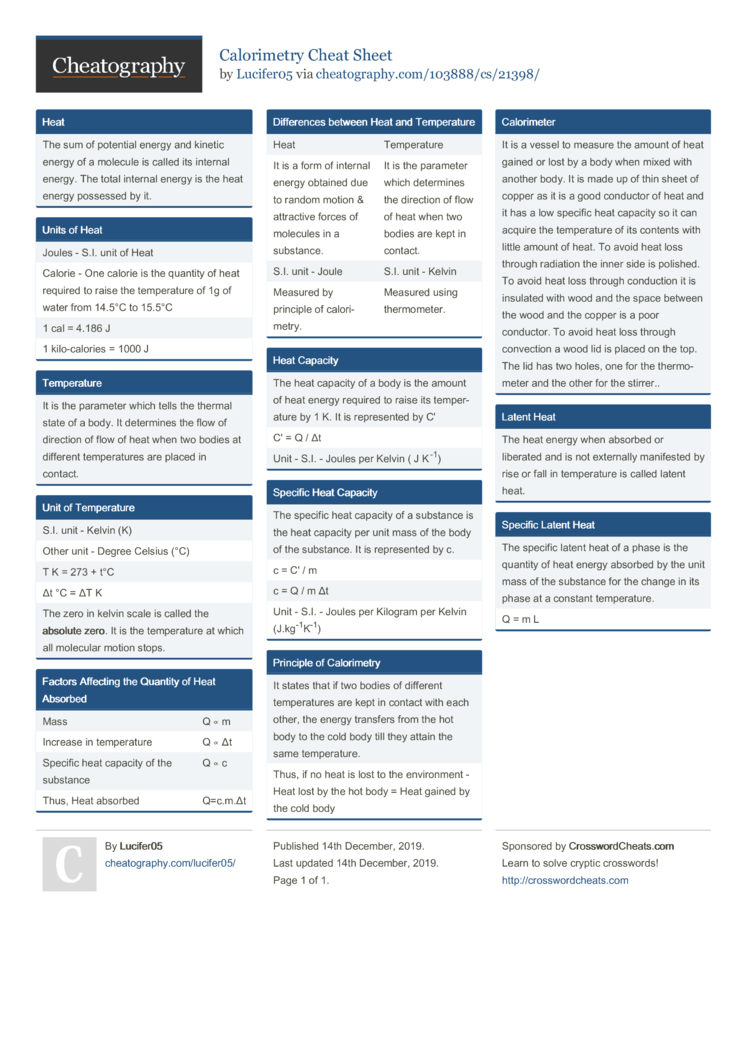# Calorimetry Cheat Sheet by Lucifer05

### Heat

 The sum of potential energy and kinetic energy of a molecule is called its internal energy. The total internal energy is the heat energy possessed by it.

### Units of Heat

 Joules - S.I. unit of Heat Calorie - One calorie is the quantity of heat required to raise the temper­ature of 1g of water from 14.5°C to 15.5°C 1 cal = 4.186 J 1 kilo-c­alories = 1000 J

### Temper­ature

 It is the parameter which tells the thermal state of a body. It determines the flow of direction of flow of heat when two bodies at different temper­atures are placed in contact.

### Unit of Temper­ature

 S.I. unit - Kelvin (K) Other unit - Degree Celsius (°C) T K = 273 + t°C Δt °C = ΔT K The zero in kelvin scale is called the absolute zero. It is the temper­ature at which all molecular motion stops.

### Factors Affecting the Quantity of Heat Absorbed

 Mass Q ∝ m Increase in temper­ature Q ∝ Δt Specific heat capacity of the substance Q ∝ c Thus, Heat absorbed Q=c.m.Δt

### Differ­ences between Heat and Temper­ature

 Heat Temper­ature It is a form of internal energy obtained due to random motion & attractive forces of molecules in a substance. It is the parameter which determines the direction of flow of heat when two bodies are kept in contact. S.I. unit - Joule S.I. unit - Kelvin Measured by principle of calori­metry. Measured using thermo­meter.

### Heat Capacity

 The heat capacity of a body is the amount of heat energy required to raise its temper­ature by 1 K. It is repres­ented by C' C' = Q / Δt Unit - S.I. - Joules per Kelvin ( J K-1)

### Specific Heat Capacity

 The specific heat capacity of a substance is the heat capacity per unit mass of the body of the substance. It is repres­ented by c. c = C' / m c = Q / m Δt Unit - S.I. - Joules per Kilogram per Kelvin (J.kg­-1­K-1)

### Principle of Calori­metry

 It states that if two bodies of different temper­atures are kept in contact with each other, the energy transfers from the hot body to the cold body till they attain the same temper­ature. Thus, if no heat is lost to the enviro­nment - Heat lost by the hot body = Heat gained by the cold body

### Calori­meter

 It is a vessel to measure the amount of heat gained or lost by a body when mixed with another body. It is made up of thin sheet of copper as it is a good conductor of heat and it has a low specific heat capacity so it can acquire the temper­ature of its contents with little amount of heat. To avoid heat loss through radiation the inner side is polished. To avoid heat loss through conduction it is insulated with wood and the space between the wood and the copper is a poor conductor. To avoid heat loss through convection a wood lid is placed on the top. The lid has two holes, one for the thermo­meter and the other for the stirrer.

### Latent Heat

 The heat energy when absorbed or liberated and is not externally manifested by rise or fall in temper­ature is called latent heat.

### Specific Latent Heat

 The specific latent heat of a phase is the quantity of heat energy absorbed by the unit mass of the substance for the change in its phase at a constant temper­ature. Q = m L1 Page
//media.cheatography.com/storage/thumb/lucifer05_calorimetry.750.jpg

PDF (recommended)